This analysis is specifically just geared towards showing the R code. I have provided some thoughts on the analysis here.

First, we will read the dataset and look at the first row.

``````si <- read.csv('filepath/to/your/csv/file')
``````##   X protest_date   alive  name gender map_listing tibetan_area
## 1 1   27-02-2009 Unknown Tapey      M       Ngaba        Ngaba
##   tibetan_region place_in_chinese original_age age Occupation   group
## 1           Amdo          Sichuan         <NA>  NA       <NA> Unknown
## 1          <NA>     <NA>             <NA> http://bit.ly/Vf0D3g
## 1 http://www.flickr.com/photos/internationaltibetnetwork/8230550078/in/set-72157630599786116/``````

We can also look at both the summary and structure of our dataset using commands `summary` and `str` respectively. This gives us an idea of the variable types we are dealing with. Using `str`, we can see that the protest date is interpreted as a non-date data type. We convert it to date-type in a new column ‘dates’. We will use the dates column to help us with our plots.

``str(si)``
``````## 'data.frame':    138 obs. of  18 variables:
##  \$ X                 : int  1 2 3 5 6 7 8 9 11 10 ...
##  \$ protest_date      : Factor w/ 110 levels "","01-12-2011",..: 97 53 50 93 6 22 22 51 89 65 ...
##  \$ alive             : Factor w/ 3 levels "No","Unknown",..: 2 1 1 3 3 1 1 1 3 1 ...
##  \$ name              : Factor w/ 132 levels "Ani Dolma","Atse",..: 97 75 122 53 29 7 32 66 11 107 ...
##  \$ gender            : Factor w/ 2 levels "F","M": 2 2 2 2 2 2 2 2 2 1 ...
##  \$ map_listing       : Factor w/ 38 levels "Achok","Amchok",..: 28 28 33 28 28 28 28 28 20 28 ...
##  \$ tibetan_area      : Factor w/ 49 levels "Achok, Labrang, Sangchu",..: 34 34 45 34 34 34 34 34 26 34 ...
##  \$ tibetan_region    : Factor w/ 3 levels "Amdo","Kham",..: 1 1 2 1 1 1 1 1 2 1 ...
##  \$ place_in_chinese  : Factor w/ 5 levels "Beijing","Gansu",..: 4 4 3 4 4 4 4 4 4 3 ...
##  \$ original_age      : Factor w/ 48 levels "","15","16","17",..: NA NA NA NA NA NA NA NA 22 NA ...
##  \$ age               : int  NA NA NA NA NA NA NA NA 35 NA ...
##  \$ Occupation        : Factor w/ 23 levels "","cattle_herder",..: NA NA NA NA NA NA NA NA 1 17 ...
##  \$ group             : Factor w/ 8 levels "Activist","Community leader",..: 8 8 8 8 8 8 8 8 8 6 ...
##  \$ family_status     : Factor w/ 47 levels "2 children ",..: NA NA NA NA NA NA NA NA NA NA ...
##  \$ children          : Factor w/ 6 levels "/","0","1","2",..: NA NA NA NA NA NA NA NA NA NA ...
##  \$ messages_slogans  : Factor w/ 55 levels ""," For less than two weeks, from November 7 to 18, Tsegyal received no treatment for his burns while being held at the local poli"| __truncated__,..: NA NA NA NA NA NA NA NA NA NA ...
##  \$ image_bitly_links : Factor w/ 125 levels "http://bit.ly/10wWBGe",..: 94 58 87 47 11 84 78 119 107 64 ...
##  \$ image_flickr_links: Factor w/ 124 levels "","http://www.flickr.com/photos/internationaltibetnetwork/11221799883/in/set-72157630599786116/",..: 96 95 94 54 93 53 92 52 51 91 ...``````
``````# Insert new column dates as 'protest_date' was not in date format
si\$dates <- as.Date(si\$protest_date, "%d-%m-%Y")
``````##   X protest_date   alive  name gender map_listing tibetan_area
## 1 1   27-02-2009 Unknown Tapey      M       Ngaba        Ngaba
##   tibetan_region place_in_chinese original_age age Occupation   group
## 1           Amdo          Sichuan         <NA>  NA       <NA> Unknown
## 1          <NA>     <NA>             <NA> http://bit.ly/Vf0D3g
## 1 http://www.flickr.com/photos/internationaltibetnetwork/8230550078/in/set-72157630599786116/
##        dates
## 1 2009-02-27``````
``````si\$years <- as.numeric(format(si\$dates, format = "%Y"))
si\$years <- as.integer(si\$years)
``````##   X protest_date   alive  name gender map_listing tibetan_area
## 1 1   27-02-2009 Unknown Tapey      M       Ngaba        Ngaba
##   tibetan_region place_in_chinese original_age age Occupation   group
## 1           Amdo          Sichuan         <NA>  NA       <NA> Unknown
## 1          <NA>     <NA>             <NA> http://bit.ly/Vf0D3g
## 1 http://www.flickr.com/photos/internationaltibetnetwork/8230550078/in/set-72157630599786116/
##        dates years
## 1 2009-02-27  2009``````
``str(si)``
``````## 'data.frame':    138 obs. of  20 variables:
##  \$ X                 : int  1 2 3 5 6 7 8 9 11 10 ...
##  \$ protest_date      : Factor w/ 110 levels "","01-12-2011",..: 97 53 50 93 6 22 22 51 89 65 ...
##  \$ alive             : Factor w/ 3 levels "No","Unknown",..: 2 1 1 3 3 1 1 1 3 1 ...
##  \$ name              : Factor w/ 132 levels "Ani Dolma","Atse",..: 97 75 122 53 29 7 32 66 11 107 ...
##  \$ gender            : Factor w/ 2 levels "F","M": 2 2 2 2 2 2 2 2 2 1 ...
##  \$ map_listing       : Factor w/ 38 levels "Achok","Amchok",..: 28 28 33 28 28 28 28 28 20 28 ...
##  \$ tibetan_area      : Factor w/ 49 levels "Achok, Labrang, Sangchu",..: 34 34 45 34 34 34 34 34 26 34 ...
##  \$ tibetan_region    : Factor w/ 3 levels "Amdo","Kham",..: 1 1 2 1 1 1 1 1 2 1 ...
##  \$ place_in_chinese  : Factor w/ 5 levels "Beijing","Gansu",..: 4 4 3 4 4 4 4 4 4 3 ...
##  \$ original_age      : Factor w/ 48 levels "","15","16","17",..: NA NA NA NA NA NA NA NA 22 NA ...
##  \$ age               : int  NA NA NA NA NA NA NA NA 35 NA ...
##  \$ Occupation        : Factor w/ 23 levels "","cattle_herder",..: NA NA NA NA NA NA NA NA 1 17 ...
##  \$ group             : Factor w/ 8 levels "Activist","Community leader",..: 8 8 8 8 8 8 8 8 8 6 ...
##  \$ family_status     : Factor w/ 47 levels "2 children ",..: NA NA NA NA NA NA NA NA NA NA ...
##  \$ children          : Factor w/ 6 levels "/","0","1","2",..: NA NA NA NA NA NA NA NA NA NA ...
##  \$ messages_slogans  : Factor w/ 55 levels ""," For less than two weeks, from November 7 to 18, Tsegyal received no treatment for his burns while being held at the local poli"| __truncated__,..: NA NA NA NA NA NA NA NA NA NA ...
##  \$ image_bitly_links : Factor w/ 125 levels "http://bit.ly/10wWBGe",..: 94 58 87 47 11 84 78 119 107 64 ...
##  \$ image_flickr_links: Factor w/ 124 levels "","http://www.flickr.com/photos/internationaltibetnetwork/11221799883/in/set-72157630599786116/",..: 96 95 94 54 93 53 92 52 51 91 ...
##  \$ dates             : Date, format: "2009-02-27" "2011-03-16" ...
##  \$ years             : int  2009 2011 2012 2011 2011 2011 2011 2011 2011 2011 ...``````

Let us take a quick look at a few plots. Part of the exploratory data analysis in relation to plots is we try to observe various variables and see if there are any hidden patterns.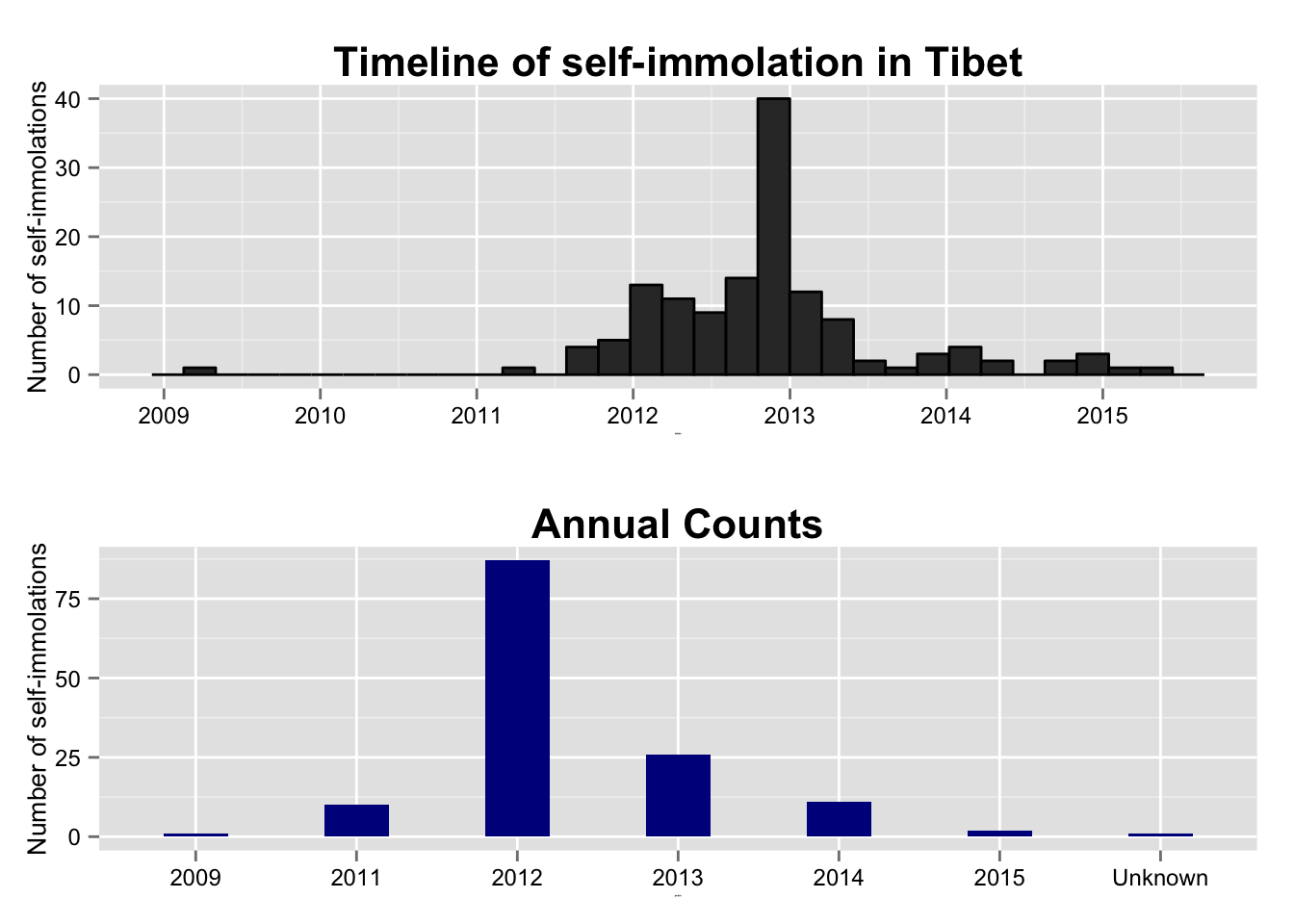``````p1 = ggplot(aes(x=dates, fill=tibetan_region), data=si) +
geom_bar() +
geom_bar(color="black", show_guide=FALSE) +
scale_x_date(labels=date_format("%Y")) +
theme(axis.title.x = element_text(size=1),
axis.title.y = element_text(size=1),
axis.text.x = element_text(size=9),
axis.text.y = element_text(size=9),
plot.title = element_text(face="bold", size=14)) +
ylab('Number of self-immolations') + guides(fill=guide_legend(title="Region")) +
labs(title = 'Regional breakdown') +
scale_fill_manual(values=c("orange", "green", "blue"))

# scale_x_date(labels=date_format("%Y-%b"), breaks="4 month") +
p2 = ggplot(aes(x=dates, fill=gender), data=si) +
geom_bar() +
geom_bar(color="black", show_guide=FALSE) +
scale_x_date(labels=date_format("%Y")) +
theme(axis.title.x = element_text(size=1),
axis.title.y = element_text(size=1),
axis.text.x = element_text(size=9),
axis.text.y = element_text(size=9),
plot.title = element_text(face="bold", size=14)) +
ylab('Number of self-immolations') + guides(fill=guide_legend(title="Gender")) +
labs(title = 'Gender breakdown')

# grid arrange
grid.arrange(p1, p2, ncol=1)``````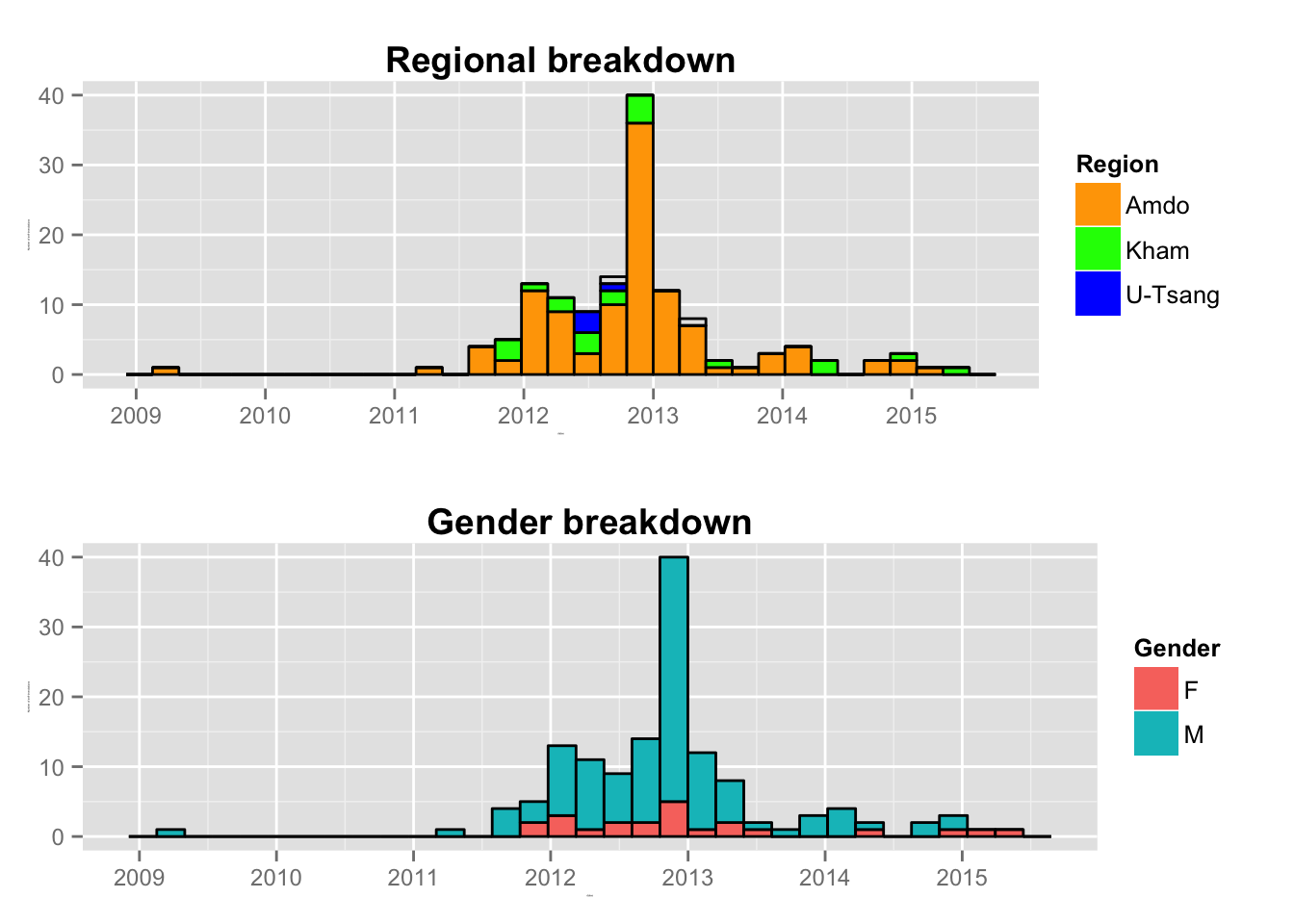``````# Age
ggplot(aes(x = age, fill=gender), data = subset(si, !is.na(age))) +
geom_bar() +
geom_bar(color="black", show_guide=FALSE) +
scale_x_continuous(limits = c(14, 70), breaks = c(15,18,21,24,27,30,35,40,50,66)) +
theme(axis.title.x = element_text(face="bold", size=14),
axis.title.y = element_text(size=13),
axis.text.x = element_text(size=11, color="black"),
axis.text.y = element_text(size=11, color="black"),
plot.title = element_text(face="bold", size=16)) +
xlab('Age') + ylab('Number of self-immolations') + guides(fill=guide_legend(title="Gender")) +
labs(title = 'Self-immolation in Tibet: Gender-Age breakdown')``````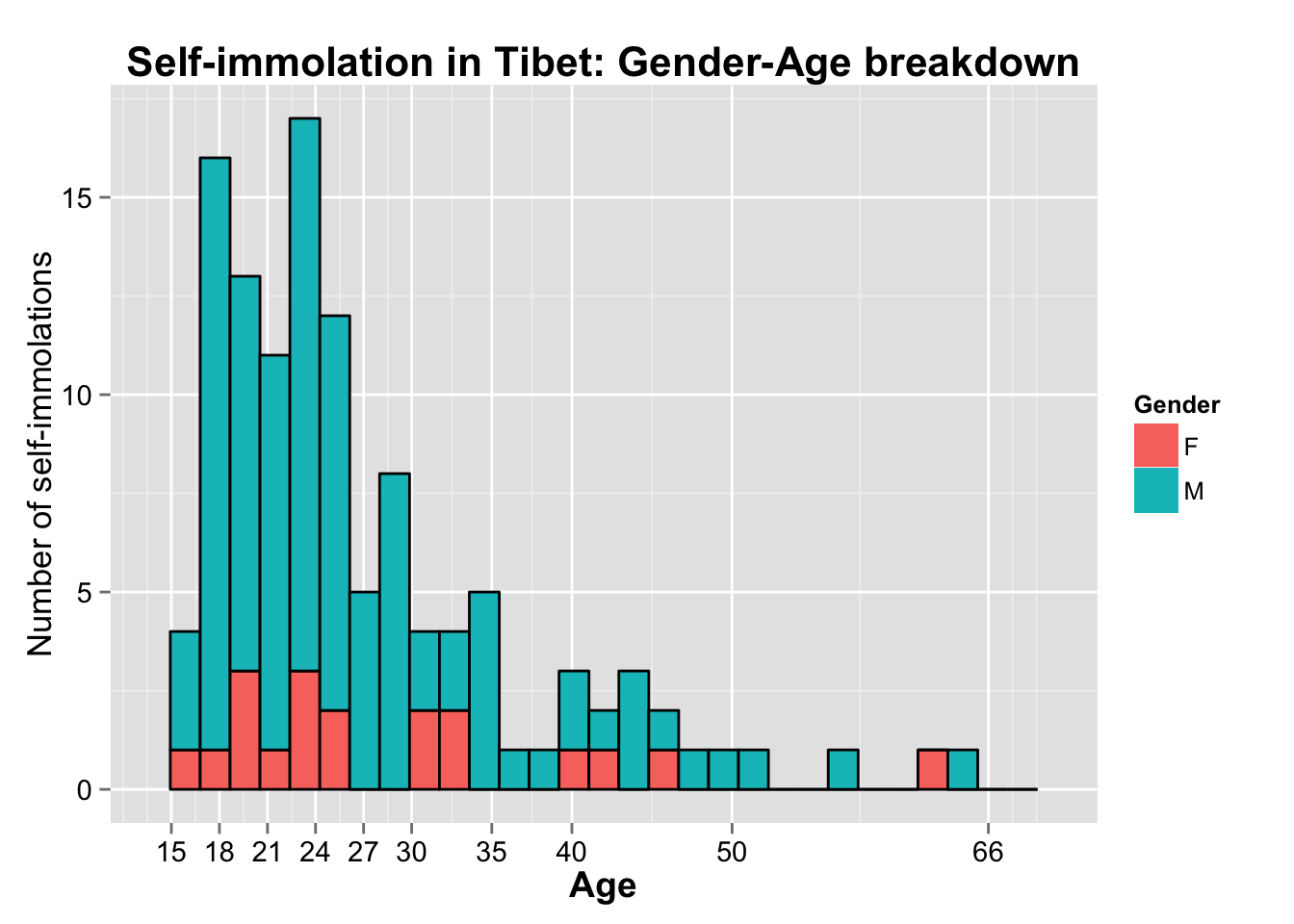Next, let’s dive deeper into this age demographic and split it based on region, gender, and occupation. Two things you notice in the occupation graph are the “unknown” and “religious ascetic” groups. The high number of unknowns reflect the challenges of collecting information from inside Tibet. It becomes imperative to ensure Tibetans inside and outside Tibet continue to make efforts to collect personal information so that we can recognize these brave individuals. The corollary to that is the Chinese military using the same information to detain and arrest the person’s family members and relatives.

As for the religious ascetic group, this group includes monks, nuns, and former religious officials.

``````# Regional age breakdown
ggplot(aes(x = age, fill=tibetan_region), data = subset(si, !is.na(age))) +
geom_bar() +
geom_bar(color="black", show_guide=FALSE) +
scale_x_continuous(limits = c(14, 70), breaks = c(15,18,21,24,27,30,35,40,50,66)) +
theme(axis.title.x = element_text(face="bold", size=14),
axis.title.y = element_text(size=14),
axis.text.x = element_text(size=11, color="black"),
axis.text.y = element_text(size=11, color="black"),
plot.title = element_text(face="bold", size=16)) +
xlab('Age') + ylab('Number of self-immolations') + guides(fill=guide_legend(title="Region")) +
labs(title = 'Self-immolation in Tibet: Regional-Age breakdown') +
scale_fill_manual(values=cbbPalette)``````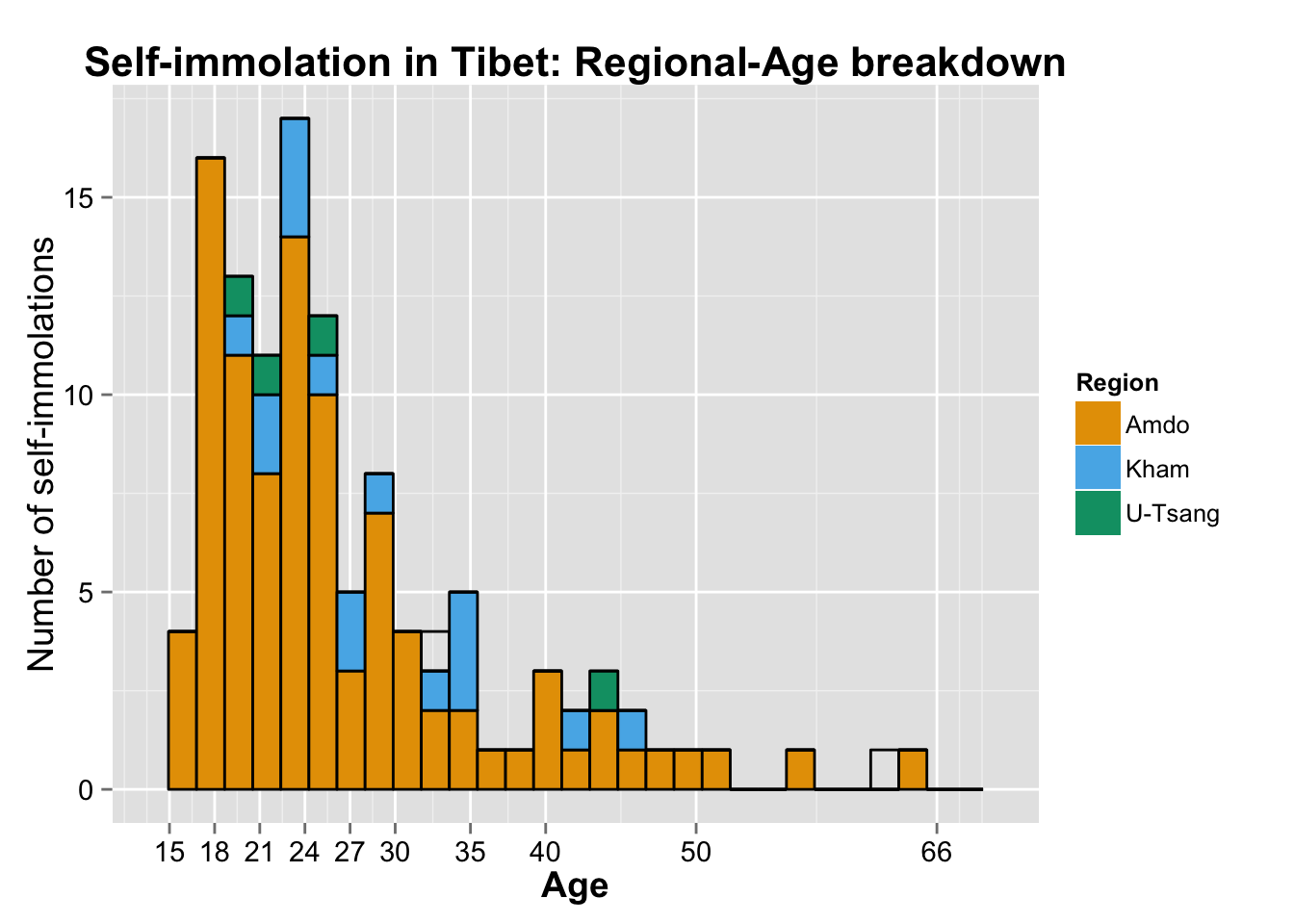``````# Region - facet grid (not wrap)
ggplot(aes(x = age, fill=gender), data = subset(si, !is.na(age))) +
geom_bar() +
geom_bar(color="black", show_guide=FALSE) +
scale_x_continuous(limits = c(14, 70), breaks = seq(15,70, 10)) +
theme(axis.title.x = element_text(face="bold", size=14),
axis.title.y = element_text(size=14),
axis.text.x = element_text(size=11, color="black"),
axis.text.y = element_text(size=11, color="black"),
plot.title = element_text(face="bold", size=16),
strip.text.y = element_text(size=10, face="bold")) +
xlab('Age') + ylab('Number of self-immolations') + guides(fill=guide_legend(title="Gender")) +
labs(title = 'Gender-Age breakdown based on Tibetan regions') +
facet_grid(tibetan_region ~ .)``````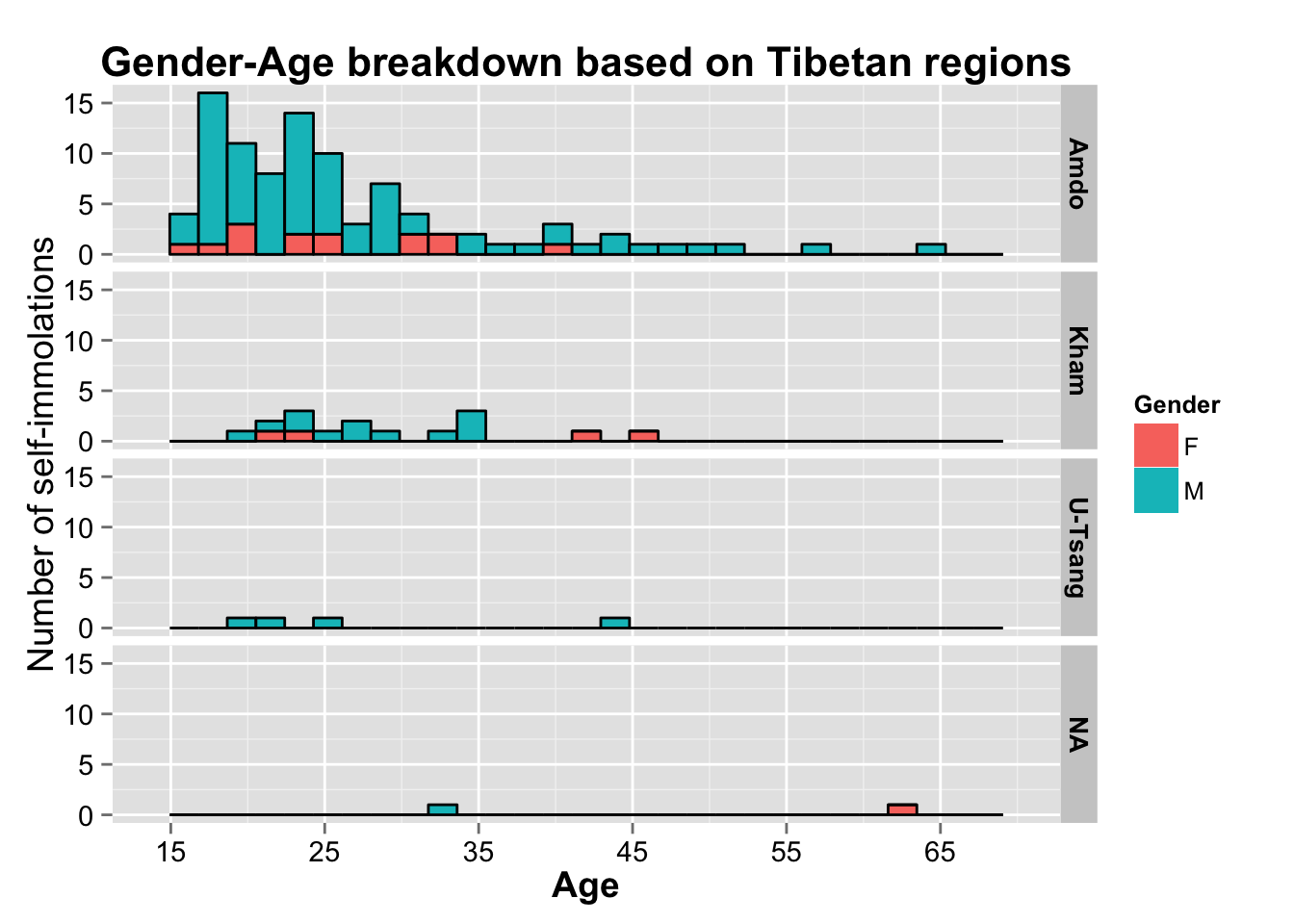``````#scale_fill_manual(values=cbbPalette) +

ggplot(aes(x = age, fill=gender), data = subset(si, !is.na(age))) +
geom_bar() +
geom_bar(color="black", show_guide=FALSE) +
scale_x_continuous(limits = c(14, 70), breaks = seq(15,70, 10)) +
theme(axis.title.x = element_text(face="bold", size=14),
axis.title.y = element_text(size=14),
axis.text.x = element_text(size=9, color="black"),
axis.text.y = element_text(size=9, color="black"),
plot.title = element_text(face="bold", size=16),
strip.text.x = element_text(size=10),
strip.text.y = element_text(size=10, face="bold")) +
xlab('Age') + ylab('Number of self-immolations') + guides(fill=guide_legend(title="Gender")) +
labs(title = 'Annual Gender-Age breakdown') +
facet_grid(tibetan_region ~ years)``````